SSC CGL Previous Year Questions: Time, Speed & Distance (Boat and Streams) - 3

# SSC CGL Previous Year Questions: Time, Speed & Distance (Boat and Streams) - 3 - SSC CGL

Test Description

## 23 Questions MCQ Test SSC CGL (Tier - 1) - Previous Year Papers (Topic Wise) - SSC CGL Previous Year Questions: Time, Speed & Distance (Boat and Streams) - 3

SSC CGL Previous Year Questions: Time, Speed & Distance (Boat and Streams) - 3 for SSC CGL 2023 is part of SSC CGL (Tier - 1) - Previous Year Papers (Topic Wise) preparation. The SSC CGL Previous Year Questions: Time, Speed & Distance (Boat and Streams) - 3 questions and answers have been prepared according to the SSC CGL exam syllabus.The SSC CGL Previous Year Questions: Time, Speed & Distance (Boat and Streams) - 3 MCQs are made for SSC CGL 2023 Exam. Find important definitions, questions, notes, meanings, examples, exercises, MCQs and online tests for SSC CGL Previous Year Questions: Time, Speed & Distance (Boat and Streams) - 3 below.
Solutions of SSC CGL Previous Year Questions: Time, Speed & Distance (Boat and Streams) - 3 questions in English are available as part of our SSC CGL (Tier - 1) - Previous Year Papers (Topic Wise) for SSC CGL & SSC CGL Previous Year Questions: Time, Speed & Distance (Boat and Streams) - 3 solutions in Hindi for SSC CGL (Tier - 1) - Previous Year Papers (Topic Wise) course. Download more important topics, notes, lectures and mock test series for SSC CGL Exam by signing up for free. Attempt SSC CGL Previous Year Questions: Time, Speed & Distance (Boat and Streams) - 3 | 23 questions in 23 minutes | Mock test for SSC CGL preparation | Free important questions MCQ to study SSC CGL (Tier - 1) - Previous Year Papers (Topic Wise) for SSC CGL Exam | Download free PDF with solutions
 1 Crore+ students have signed up on EduRev. Have you?
SSC CGL Previous Year Questions: Time, Speed & Distance (Boat and Streams) - 3 - Question 1

### A train 270 metre long is running at a speed of 36 km per hour, then it will cross a bridge of length 180 metres in:   (SSC Sub. Ins. 2013)

Detailed Solution for SSC CGL Previous Year Questions: Time, Speed & Distance (Boat and Streams) - 3 - Question 1

Speed of train = 36 kmph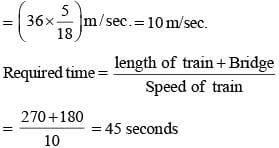SSC CGL Previous Year Questions: Time, Speed & Distance (Boat and Streams) - 3 - Question 2

### On a journey across Kolkata, a taxi averages 50 km per hour for 50% of the distance. 40 km per hour for 40% of it and 20 km per hour for the remaining. The average speed in km/hour, for the whole journey is:    (SSC Sub. Ins. 2013)

Detailed Solution for SSC CGL Previous Year Questions: Time, Speed & Distance (Boat and Streams) - 3 - Question 2

Total distance = 100 km.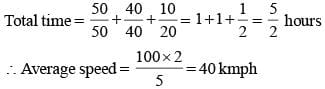SSC CGL Previous Year Questions: Time, Speed & Distance (Boat and Streams) - 3 - Question 3

### Two trains 108 m and 112 m in length are running towards each other on the parallel lines at a speed of 45 km/hr and 54 km/hr respectively. To cross each other after they meet, it will take   (SSC Multitasking 2013)

Detailed Solution for SSC CGL Previous Year Questions: Time, Speed & Distance (Boat and Streams) - 3 - Question 3

Relative speed = (45 + 54) 99km/hr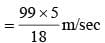Distance covered in crossing each other = (108 + 112) = 220m
Required time =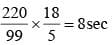SSC CGL Previous Year Questions: Time, Speed & Distance (Boat and Streams) - 3 - Question 4

Walking at a speed of 5 km/hr, a man reaches his office 6 minutes late. Walking at 6 km/hr, he reaches there 2 minutes early. The distance of his office is   (SSC Multitasking 2013)

Detailed Solution for SSC CGL Previous Year Questions: Time, Speed & Distance (Boat and Streams) - 3 - Question 4

Let the required distance be x km.
Difference in the times taken at two speeds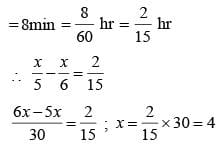Hence, the required distance is 4km.

SSC CGL Previous Year Questions: Time, Speed & Distance (Boat and Streams) - 3 - Question 5

The minute hand of a big wall-clock is 35 cm long. Taking π = 22/7, length of the arc, its extremity moves in 18 seconds is:             (SSC CHSL 2012)

Detailed Solution for SSC CGL Previous Year Questions: Time, Speed & Distance (Boat and Streams) - 3 - Question 5

Length of arc in 18 seconds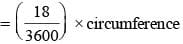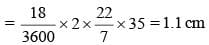SSC CGL Previous Year Questions: Time, Speed & Distance (Boat and Streams) - 3 - Question 6

A train 100 metres long meets a man going in opposite Directions at 5 km/hr and passes him in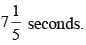What is the speed of the train in km/hr?   (SSC CHSL 2012)

Detailed Solution for SSC CGL Previous Year Questions: Time, Speed & Distance (Boat and Streams) - 3 - Question 6

Let speed of train = x km/hr
Distance travelled by train
= Relative speed of train × Time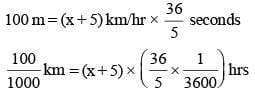⇒  x + 5 = 50
∴ x  = 45 km/hr

SSC CGL Previous Year Questions: Time, Speed & Distance (Boat and Streams) - 3 - Question 7

A man walks ‘a‘ km in ‘b’ hours. The time taken to walk 200 metres is:   (SSC CHSL 2012)

Detailed Solution for SSC CGL Previous Year Questions: Time, Speed & Distance (Boat and Streams) - 3 - Question 7

1 km = 1000 m
D = S × T
a km = S × b hr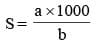Now, D = 200 m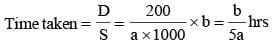SSC CGL Previous Year Questions: Time, Speed & Distance (Boat and Streams) - 3 - Question 8

A man walks a certain distance and rides back taking a total time of 37 minutes. He could walk both ways in 55 minutes.
How long would he take to ride both ways?   (SSC Sub. Ins. 2012)

Detailed Solution for SSC CGL Previous Year Questions: Time, Speed & Distance (Boat and Streams) - 3 - Question 8

To walk both ways, duration = 55 minutes
∴ To walk one way, duration = 55/2 minutes
To walk one way + To ride one way = 37 minutes
∴ To ride both ways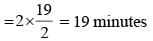SSC CGL Previous Year Questions: Time, Speed & Distance (Boat and Streams) - 3 - Question 9

A car covers four successive 6 km stretches at speeds of 25 kmph, 50 kmph, 75 kmph and 150 kmph respectively. Its average speed over this distance is    (SSC CGL 2nd Sit. 2012)

Detailed Solution for SSC CGL Previous Year Questions: Time, Speed & Distance (Boat and Streams) - 3 - Question 9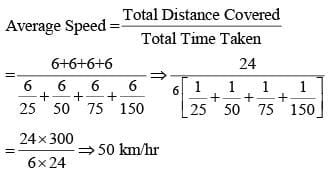SSC CGL Previous Year Questions: Time, Speed & Distance (Boat and Streams) - 3 - Question 10

A ship is moving at a speed of 30 km/hr. To know the depth of the ocean beneath it, it sends a radiowave which travels at a speed 200 m/s. The ship receives the signal after it has moved 500 m. The depth of the ocean is   (SSC CGL 1st Sit. 2012)

Detailed Solution for SSC CGL Previous Year Questions: Time, Speed & Distance (Boat and Streams) - 3 - Question 10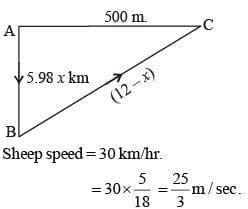Time taken by the sheep to travel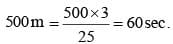Distance travelled by the signal = 60 × 200 = 12000 m. = 12 km.
Let the depth of the sea is x km.
then from ΔABC, AB  = x km, BC = (12 – x) km.
AC = 500m, = 0.5km.
Now, (BC)2 = (AB)2 + (AC)2
(12 – x)2 = x2 + (0.5)2.
144 –  24x = 0.25.
x = 6km.

SSC CGL Previous Year Questions: Time, Speed & Distance (Boat and Streams) - 3 - Question 11

With average speed of 40 km/hour, a train reaches its’ destination in time. If it goes with an average speed of 35 km/hour, it is late by 15 minutes. The total journey is    (SSC CGL 1st Sit. 2012)

Detailed Solution for SSC CGL Previous Year Questions: Time, Speed & Distance (Boat and Streams) - 3 - Question 11

If the total length of journey be x km, then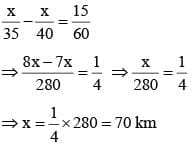SSC CGL Previous Year Questions: Time, Speed & Distance (Boat and Streams) - 3 - Question 12

A train overtakes two persons who are walking in the same direction in which the train is running, at the rate of 2 kmph and 4 kmph and passes them completely in 9 and 10 seconds respectively. The length of the train (in metres):   (SSC CGL 2nd Sit. 2012)

Detailed Solution for SSC CGL Previous Year Questions: Time, Speed & Distance (Boat and Streams) - 3 - Question 12

Let the length of train be x km and its speed by y kmph.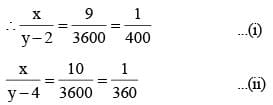By dividing equation  (i) by (ii),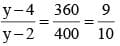⇒ 10y – 40 = 9y – 18
⇒ y = 40 – 18 = 22
From equation (i),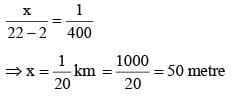Alternate Method:
Let Speed of train be x km/h
According to question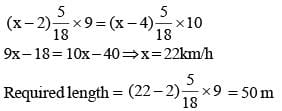SSC CGL Previous Year Questions: Time, Speed & Distance (Boat and Streams) - 3 - Question 13

A thief is noticed by a policeman from a distance of 200 m. The thief starts running and the policeman chases him. The thief and the policeman run at the rate of 10 km and 11 km per hour respectively. The distance (in metres) between them after 6 minutes is    (SSC CGL 1st Sit. 2012)

Detailed Solution for SSC CGL Previous Year Questions: Time, Speed & Distance (Boat and Streams) - 3 - Question 13

Relative speed = 11 – 10 = 1 kmph
Distance covered in 6 minutes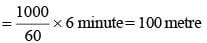∴ Remaining distance = 200 – 100 = 100 metre

SSC CGL Previous Year Questions: Time, Speed & Distance (Boat and Streams) - 3 - Question 14

Walking at 5 km/hr a student reaches his school from his house 15 minutes early and walking at 3 km/hr he is late by 9 minutes. What is the distance between his school and his house?   (SSC CGL  2nd Sit. 2011)

Detailed Solution for SSC CGL Previous Year Questions: Time, Speed & Distance (Boat and Streams) - 3 - Question 14

Let the required distance be x km.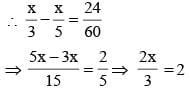⇒ 2x = 2 × 3 ⇒  x = 3 km

SSC CGL Previous Year Questions: Time, Speed & Distance (Boat and Streams) - 3 - Question 15

Walking at 6th/7 of his usual speed a man is 25 minutes too late. His usual time to cover this distance is    (SSC CGL 2nd Sit. 2011)

Detailed Solution for SSC CGL Previous Year Questions: Time, Speed & Distance (Boat and Streams) - 3 - Question 15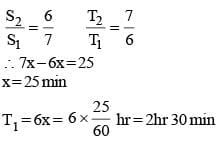SSC CGL Previous Year Questions: Time, Speed & Distance (Boat and Streams) - 3 - Question 16

A student goes to school at the rate of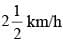and reaches 6 minutes late. If he travels at the speed of 3 km/h. he is 10 minutes early. The distance (in km) between the school and his house is   (SSC CGL 1st Sit. 2011)

Detailed Solution for SSC CGL Previous Year Questions: Time, Speed & Distance (Boat and Streams) - 3 - Question 16

Let the required distance be x km.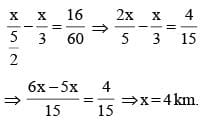SSC CGL Previous Year Questions: Time, Speed & Distance (Boat and Streams) - 3 - Question 17

A man can row 6 km/h in still water. If the speed of the current is 2 km/h, it takes 3 hours more in upstream than in the down– stream for the same distance. The distance is    (SSC CGL 1st Sit. 2011)

Detailed Solution for SSC CGL Previous Year Questions: Time, Speed & Distance (Boat and Streams) - 3 - Question 17

Speed of current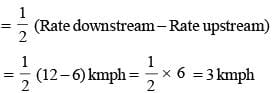SSC CGL Previous Year Questions: Time, Speed & Distance (Boat and Streams) - 3 - Question 18

A boatman rows 1 km in 5 minutes, along the stream and 6 km in 1 hour against the stream. The speed of the stream is    (SSC CGL 2nd Sit. 2010)

Detailed Solution for SSC CGL Previous Year Questions: Time, Speed & Distance (Boat and Streams) - 3 - Question 18

Speed of current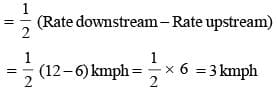SSC CGL Previous Year Questions: Time, Speed & Distance (Boat and Streams) - 3 - Question 19

A train, 240 m long crosses a man walking along the line in opposite direction at the rate of 3 kmph in 10 seconds. The speed of the train is    (SSC CGL 2nd Sit. 2010)

Detailed Solution for SSC CGL Previous Year Questions: Time, Speed & Distance (Boat and Streams) - 3 - Question 19

Let train speed be x
relative speed = (x + 3) kmph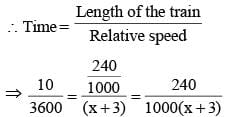⇒ x + 3 = 86.4 ⇒ x = 83.4 kmph

SSC CGL Previous Year Questions: Time, Speed & Distance (Boat and Streams) - 3 - Question 20

In a 100m race, Kamal defeats Bimal by 5 seconds. If the speed of Kamal is 18 Kmph, then the speed of Bimal is   (SSC CGL 2nd Sit. 2010)

Detailed Solution for SSC CGL Previous Year Questions: Time, Speed & Distance (Boat and Streams) - 3 - Question 20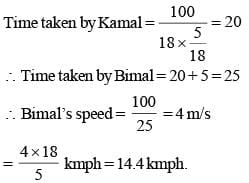SSC CGL Previous Year Questions: Time, Speed & Distance (Boat and Streams) - 3 - Question 21

A train, 300 m long, passed a man, walking along the line in the same direction at the rate of 3 km/hr in 33 seconds. The speed of the train is    (SSC CGL 1st Sit. 2010)

Detailed Solution for SSC CGL Previous Year Questions: Time, Speed & Distance (Boat and Streams) - 3 - Question 21

If the speed of the train be x kmph, then relative speed = (x – 3) kmph.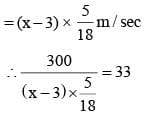⇒ 5400 = 33 × 5 (x – 3) ⇒ 360 = 11 (x – 3)
⇒ 11x – 33 = 360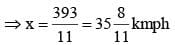SSC CGL Previous Year Questions: Time, Speed & Distance (Boat and Streams) - 3 - Question 22

By walking at 3/4 of his usual speed, a man reaches his office 20 minutes later than his usual time. The usual time taken by him to reach his office is    (SSC CGL 1st Sit. 2010)

Detailed Solution for SSC CGL Previous Year Questions: Time, Speed & Distance (Boat and Streams) - 3 - Question 22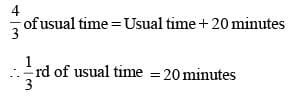∴ Usual time = 20 x 3 = 60 minutes

SSC CGL Previous Year Questions: Time, Speed & Distance (Boat and Streams) - 3 - Question 23

Buses start from a bus terminal with a speed of 20 km/hr at intervals of 10 minutes. What is the speed of a man coming from the opposite direction towards the bus terminal if he meets the buses at intervals of 8 minutes?    (SSC CGL 1st Sit. 2010)

Detailed Solution for SSC CGL Previous Year Questions: Time, Speed & Distance (Boat and Streams) - 3 - Question 23

Distance covered in 10 minutes at 20kmph = distance covered in 8 minutes at (20 + x) kmph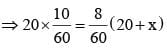⇒ 200 = 160 + 8x
⇒ 8x = 40
⇒ x = 40/8 = 5 kmph.

## SSC CGL (Tier - 1) - Previous Year Papers (Topic Wise)

250 tests
Information about SSC CGL Previous Year Questions: Time, Speed & Distance (Boat and Streams) - 3 Page
In this test you can find the Exam questions for SSC CGL Previous Year Questions: Time, Speed & Distance (Boat and Streams) - 3 solved & explained in the simplest way possible. Besides giving Questions and answers for SSC CGL Previous Year Questions: Time, Speed & Distance (Boat and Streams) - 3, EduRev gives you an ample number of Online tests for practice

250 tests